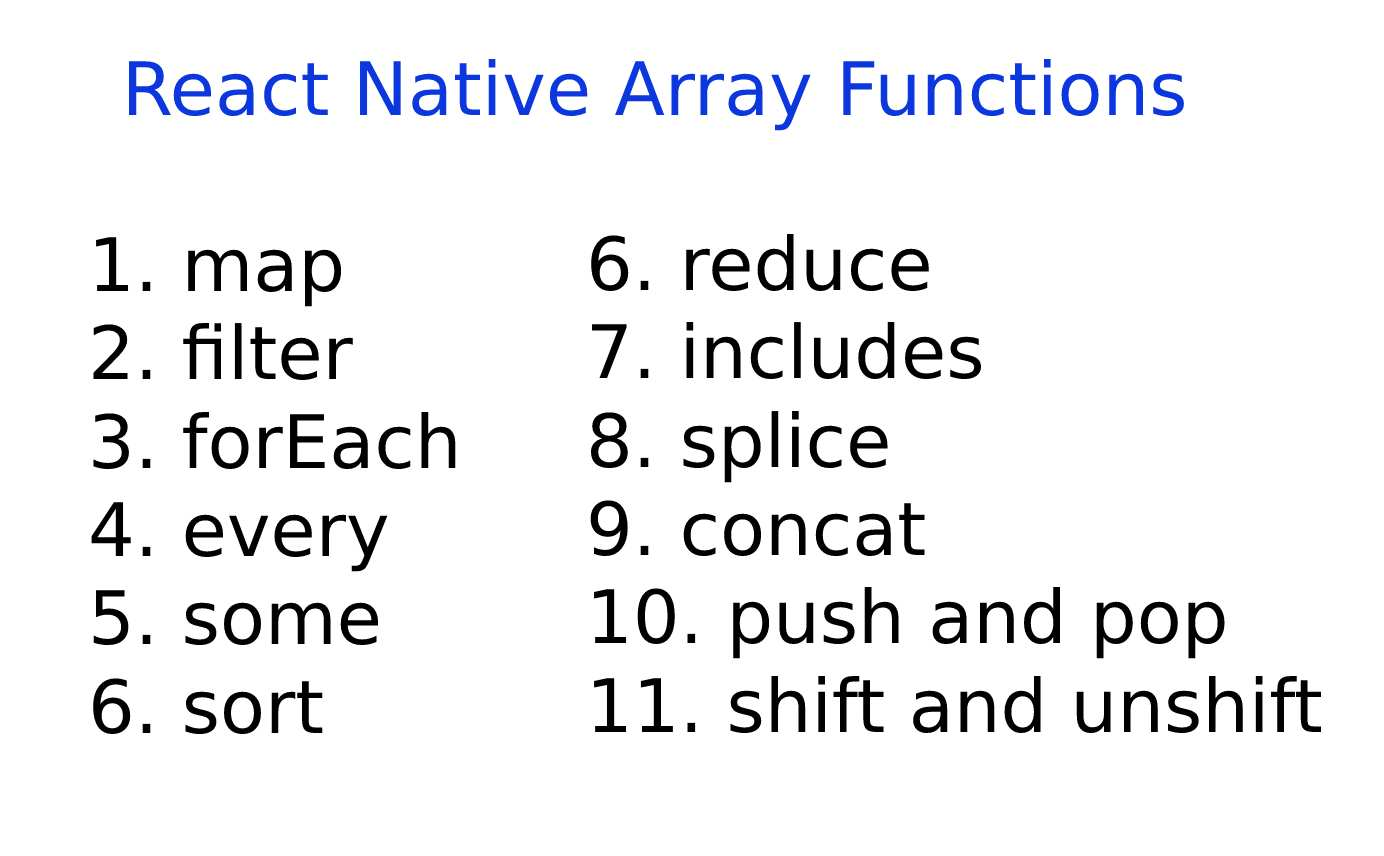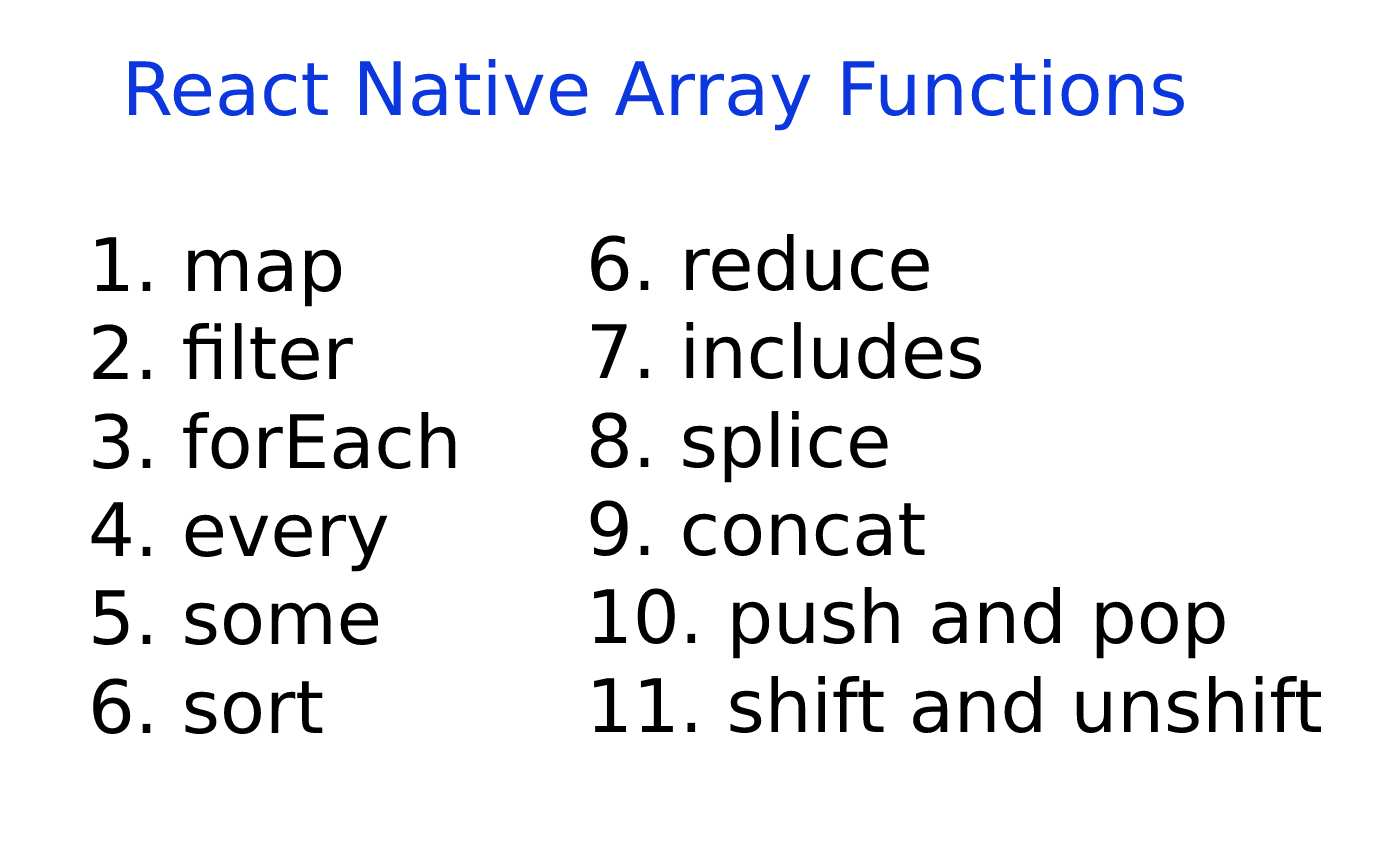Home » React-native » React Native Array Functions

# React Native Array FunctionsIn this post, we are going to learn about Javascript array functions that every React native developer should know. developer should use these functions to write robust, reusable & scalable code to create cross-platform applications. By using these functions developers can reduce time and code and create high-performance applications so let’s start with examples.### Here’s the list of javascript array functions

• map
• filter
• forEach
• every
• some
• sort
• reduce
• includes
• splice
• concet
• push and pop
• shift and unshift

### 1. map

This function creates a new array from the result of the callback function. This is the most common function used in React Native to render the list of elements it also returns the index of each element.

```var array = [2, 4, 6];
var newArr = array.map((item, index)=> item * 2)
//Result newArr = [4, 8, 12]
```

Also, you can use map() function to render a list inside the render function

```function DashboardScreen({route, navigation}) {

const renderFunction = (item, index) => {
return (
<View key={index}><Text>item</Text></View>
);
}

return(
<View style={{flex:1}}>
{dashboardReducer.dashboardResponseData.collections.map(
(item, index) => renderFunction(item, index),
)}
</View>
);

}```

### 2. filter

This filter() method will create a new array for each element that passes the test condition set in the callback function.

```var array   = [10, 20, 30, 40, 50, 60, 70, 80, 90, 100]
var list = array.filter(item => item % 2 === 10 ) // ```

### 3. forEach

This function call once for each element in the array.

```var list = [1, 2, 3, 4, 5, 6, 7, 8, 9, 10]
list.forEach((item, index) => console.log(item))```

Output:

```1
2
3
4
5
6
7
8
9
10```

### 4. every

The every() method returns a bool value if all elements in the array pass the test set in the callback function.

```var list = [10, 20, 30, 40, 50, 60, 70, 80, 90]
list.every(item => item < 100)  //It will return true

var list = [10, 20, 30, 40, 50, 60, 70, 80, 90, 100]
list.every(item => item < 100)  //It will return false```

### 5. some

The some() method returns true if at least one element in the array passes the test condition set in the callback function.

```var list = [10, 20, 30, 40, 50, 60, 70, 80, 90]
list.some(item => item % 2 === 10) //It will return true

var list = [10, 30, 40, 50, 60, 70, 80, 90, 100]
list.some(item => item % 2 === 10) //It will return false```

### 6. sort

sort() method sorts the elements in the array and returns a sorted array. we have to provide a custom comparator to sort the method because it first converts elements into strings and then sorts. let’s understand the following example

```let list = [40, 20, 10, 1000, 200]

let customComparator = (a, b) => a - b

list.sort(customComparator)
console.log(list) // prints [10, 20, 40, 200, 1000]```

### 7. reduce

As per name reduce it will reduce the number of values of the array to one single value. let’s understand with an example. we have an array of numbers [10, 20, 30, 40, 50], and we will get the sum on the array as follows

```var array = [10, 20, 30, 40, 50];
var sum = array.reduce((prevValue, currentValue) => prevValue + currentValue, 0);
//It will return sum = 150```

if we pass 10 in 0 then reduce function will take 10 as a first prevValue and add in currentValue in the array that is 10. so the output will be 160.

### 8. includes

includes function will return true if the element exists in the array.

```var array = ['first', 'second', 'third'];
console.log(array.includes('second')) // true
console.log(array.includes('forth')) // false```

### 9. splice

The function allows us to add, modify & remove elements in the array. it has the following 3 parameters

1. index
2. delete count

1. Add a new element to the array. Delete count will be 0 in this case.

```var array = ['first', 'second', 'third'];
array.splice(1, 0, 'forth') // ['first', 'forth', 'second', 'third'];```

2. Removing the 1st position element from an array.

```var array = ['first', 'forth', 'second', 'third'];
array.splice(1, 1); // ['first', 'second', 'third'];```

3. Replace element in the array

```var array = ['first', 'forth', 'second', 'third'];
array.splice(1, 1, 'fifth'); // ['first', 'fifth', 'second', 'third'];```

```var array = ['first', 'second', 'third'];
array.splice(3, 0, 'forth', 'fifth') // ['first', 'second', 'third', 'forth', 'fifth'];```

### 10. concat

This function uses to add data to the existing array. also, use to merge two arrays. note that this will not change the existing array it will return a new array.

```var array = ['first', 'second', 'third'];
var new = 'forth';
var newArray = array.concat(new) // ['first', 'second', 'third', 'forth']```

### 11. push and pop

1. The push method is used to add one or more elements at the end of the existing array & return a new array

```var array = ['first', 'second', 'third'];
array.push('forth');  //['first', 'second', 'third', 'forth']
var newArray = array.push('fifth', 'sixth'); //['first', 'second', 'third', 'forth', 'fifth', 'sixth']```

2. pop method removes only the last element in the array.

`array.pop(); //['first', 'second', 'third', 'forth', 'fifth']`

### 12. shift and unshift

shift method removes the first element of the array and returns the removed array

```var array = ['first', 'second', 'third'];
array.shift() //['second', 'third']```

unshift method adds a new element at the start of the array and returns the removed array

```var array = ['first', 'second', 'third'];
array.unshift('first', 'forth') //['first', 'forth', 'second', 'third']```

These are the React Native Array Functions. Hope this post will help you.

Thank you 🙂